Next: 2.8 General Solving Procedure Up: 2. Finite Element Method Previous: 2.6 Newton Methods

# 2.7 Assembling

For a problem described by a system of PDEs a local matrix for each element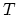of the discretization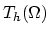, nucleus, is constructed. This nucleus is integrated into the general matrix of the system through a process called assembling. In this section the assembling algorithm applied in numerical schemes of problems discussed in Chapters 3 and 4 is derived.

The Jacobi matrix needed for the Newton method in the case of a finite element discretization is,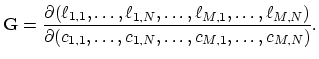(2.40)

In the further text we will call this Jacobi matrix general matrix. The inputs of the general matrix are calculated according to,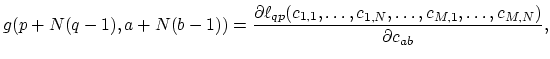(2.41)

where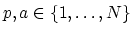,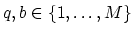, and the discrete operators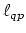are calculated as integrals over the discretization.
The calculation of the general matrix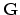by the inner product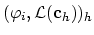over the whole discretisized domainis rarely utilized in computer programs. A common approach is to construct the matrixby assembling it out of the nucleus matrix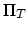which is calculated for each element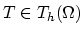,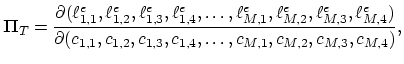(2.42)

with the inputs,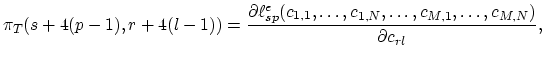(2.43)

whereand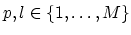.

The basic nodal function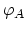, defined at the arbitrary point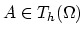is non-zero only on the patch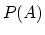. Furthermorecan be represented as,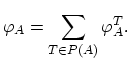(2.44)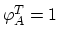at the pointand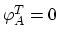at three other points of the tethraedal element.

The discrete operators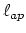obtained by testing of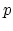-th equation of the system with the basic nodal functionis,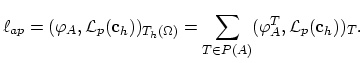(2.45)

The partial derivative of (2.45) with respect to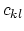is,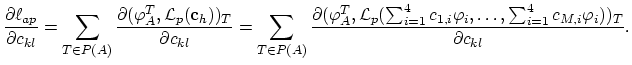(2.46)

Obviously in (2.46)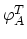is equal to one of the basic nodal functions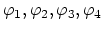at the element. Furthermore, the partial derivative is non-zero only if thestays for one nodal value of.

We now define the operator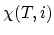,,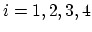, which assigns a single global index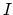to every local index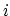of vertex belonging to the tethraedal element. The inverse function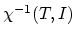is also well-defined.

From (2.41), (2.43), and (2.46) we have,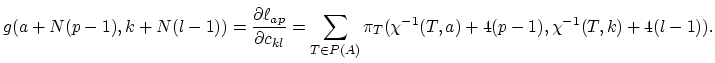(2.47)

The assembling process consists of calculating of nucleus matrix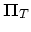for each element of the meshand building the inputs of the global matrixas the sum on the right side of the equation (2.47). For the implementation of the assembling algorithm into software tools the following algorithm is used,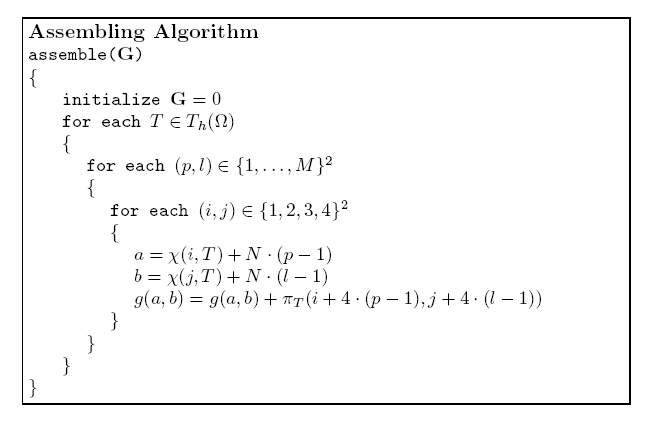At the end of the assembling process the general matrixcontains the values given by (2.40).Next: 2.8 General Solving Procedure Up: 2. Finite Element Method Previous: 2.6 Newton Methods

H. Ceric: Numerical Techniques in Modern TCAD